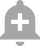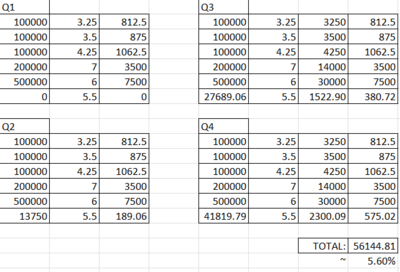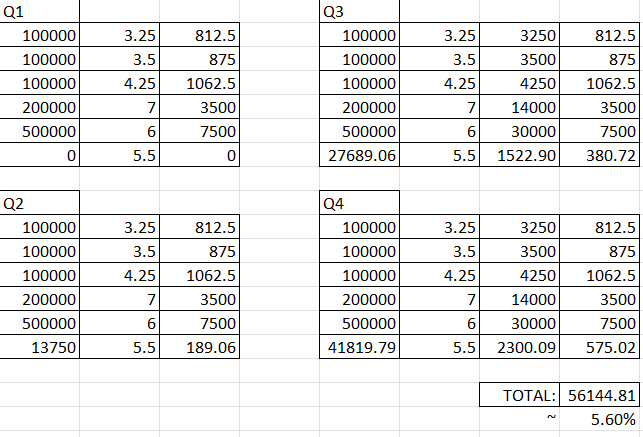# calculation of interest rates

• 485
• 15
•• Last Comment
kukdookoo
Friends most banks give interest rates slab wise in savings account, this is just an example i dont know how this bank is
https://www.dbs.com/digibank/in/banking/account...
now suppose if one is keeping balance till 2 lakhs or till 5 lakhs in this case then what will be the overall interest rate that he will be getting? Want to understand how its calculated.

@Ash-D @Rajkotian @Faug @DeciDeaim @Jarvis.-. @Sam702

Disclaimer
We are not SEBI/IRDA registered. The information provided herein is for education purposes only. We will not be responsible for any of your profit/loss with this channel's suggestions. Consult your financial advisor before making any decisions.
8 Dimers
• Sort By

So for 0-1l, it will be in that slab, for 1l-2l in next slab. You will get 3.25% on 1l and 3.5% on the second 1l.

Its credited quarterly for savings usually so the interest will also earn as per the slab

Generally banks calculate savings interest rate on incremental amount.

If interest slab is as follows :

Up to Rs 1 lakh 3.25%

Above Rs 1 lakh and up to Rs.2 Lakhs 3.50%

and you have put 2 lakh in savings

then first 1 lakh will get 3.25% and remaining 1 lakh will get 3.5%.

hope this helps.

You may calculate on your own for more balance.

Watcher wrote:

Generally banks calculate savings interest rate on incremental amount.

If interest slab is as follows :

Up to Rs 1 lakh 3.25%

Above Rs 1 lakh and up to Rs.2 Lakhs 3.50%

and you have put 2 lakh in savings

then first 1 lakh will get 3.25% and remaining 1 lakh will get 3.5%.

hope this helps.

You may calculate on your own for more balance.

i understand this but my question is say one has 2 lakhs, so interest of 3.25 and 3.50 will apply on each lakh. What is the overall interest, 3.25+3.50%/2 ? What if there are more slabs like 2-5 lakhs, 5-10 lakhs etc.? + @legend101

For 2 lakhs:

Up to Rs 1 lakh - 3.25%

Above Rs 1 lakh and up to Rs.2 Lakh - 3.5%

So 1L x 3. 25% = 3,250; and

2L - 1L = 1L x 3.5% = 3,500

3,250 + 3,500 = 6750/-

Same for 5 Lakh. Increment interest. It's same with many banks. I don't see what is the confusion here.

Expand
kukdookoo wrote:
i understand this but my question is say one has 2 lakhs, so interest of 3.25 and 3.50 will apply on each lakh. What is the overall interest, 3.25+3.50%/2 ? What if there are more slabs like 2-5 lakhs, 5-10 lakhs etc.? + @legend101
Overall interest for 1 quarter will be 3250/4=812.5 + 3500/4 = 875

To calculate the effective interest rate for a balance of Rs 10 lakhs, we need to take into account the different interest rates applied to different slabs of the balance.

First, we need to calculate the interest earned on each slab:

- Up to Rs 1 lakh: 3.25% interest = Rs 3,250

- Rs 1 lakh - Rs 2 lakhs: 3.50% interest on Rs 1 lakh = Rs 3,500

- Rs 2 lakhs - Rs 3 lakhs: 4.25% interest on Rs 1 lakh = Rs 4,250

- Rs 3 lakhs - Rs 4 lakhs: 7.00% interest on Rs 1 lakh = Rs 7,000

- Rs 4 lakhs - Rs 5 lakhs: 7.00% interest on Rs 1 lakh = Rs 7,000

- Rs 5 lakhs - Rs 10 lakhs: 6.00% interest on Rs 5 lakhs = Rs 30,000

Total interest earned = Rs 54,000

To calculate the effective interest rate, we divide the total interest earned by the balance and multiply by 100:

Effective interest rate = (Total interest earned / Balance) x 100

= (Rs 54,000 / Rs 10 lakhs) x 100

= 5.4%

Therefore, the effective interest rate for a balance of Rs 10 lakhs is 5.4%.

- ChatGPT

Expand
kukdookoo wrote:
i understand this but my question is say one has 2 lakhs, so interest of 3.25 and 3.50 will apply on each lakh. What is the overall interest, 3.25+3.50%/2 ? What if there are more slabs like 2-5 lakhs, 5-10 lakhs etc.? + @legend101
No . My understanding is the calculation will be as such.

3.25% of 1L + 3.5% of 1L

If it is 5L, then

3.25% of 1L + 3.5% of 4L and so on.
Expand
legend101 wrote:
Overall interest for 1 quarter will be 3250/4=812.5 + 3500/4 = 875
How much that comes out to be in % of the total amount? + @Fanil
Expand
kukdookoo wrote:
How much that comes out to be in % of the total amount? + @Fanil
3.375 annual

Interest on savings account = Daily balance x Rate of interest x (No. of days/365)

Assuming your account have 2.5 Lakh for 30 days then it will be calculated as follows

Interest on savings account = 100000 x (3.25/100) x (30/365) + 100000 x (3.5/100) x (30/365) + 50000 x (4.25/100) x (30/365)

I don't think there will be any fixed formula to calculate the effective interest rate in such cases, as it depends on the amount deposited in the account.

Here's a sample calculation for Rs. 10 Lac, assuming no further deposits or withdrawals.The interest in deposited every quarter, if they do deposit it every month, then the effective rate will be around 7.7%

Rajkotian wrote:

I don't think there will be any fixed formula to calculate the effective interest rate in such cases, as it depends on the amount deposited in the account.

Here's a sample calculation for Rs. 10 Lac, assuming no further deposits or withdrawals.The interest in deposited every quarter, if they do deposit it every month, then the effective rate will be around 7.7%

Yes withdrawals and interest will make it a different case but if we just consider amounts till 10lacs, ignore withdrawals and interest for a second, then the overall interest is way less than inflation.
Expand
kukdookoo wrote:
Yes withdrawals and interest will make it a different case but if we just consider amounts till 10lacs, ignore withdrawals and interest for a second, then the overall interest is way less than inflation.

Yes, Can't beat the inflation by just putting the money into the Savings account/FD 😢

Expand
Rajkotian wrote:

Yes, Can't beat the inflation by just putting the money into the Savings account/FD 😢

Yaar inflation ke thoda bahut paas hota to bhi chaltaHottest Discussions
207° India Women win Asian Games gold on cricket debutBAT_MAN
6
157° Discovered Paytm Bank's cancelled cheque optiongischethans
6
1000° Help me - HDFC charges 8k finance charge for 700rs missed payment on Tata neu infinity cc.KartikDD98
75
249° New Discount Brokers - Share.market by PhonePe & Sky by HDFCOriginal
24
294° SBI Card on BBPS (Loot Trick)abhishek012
37
View all